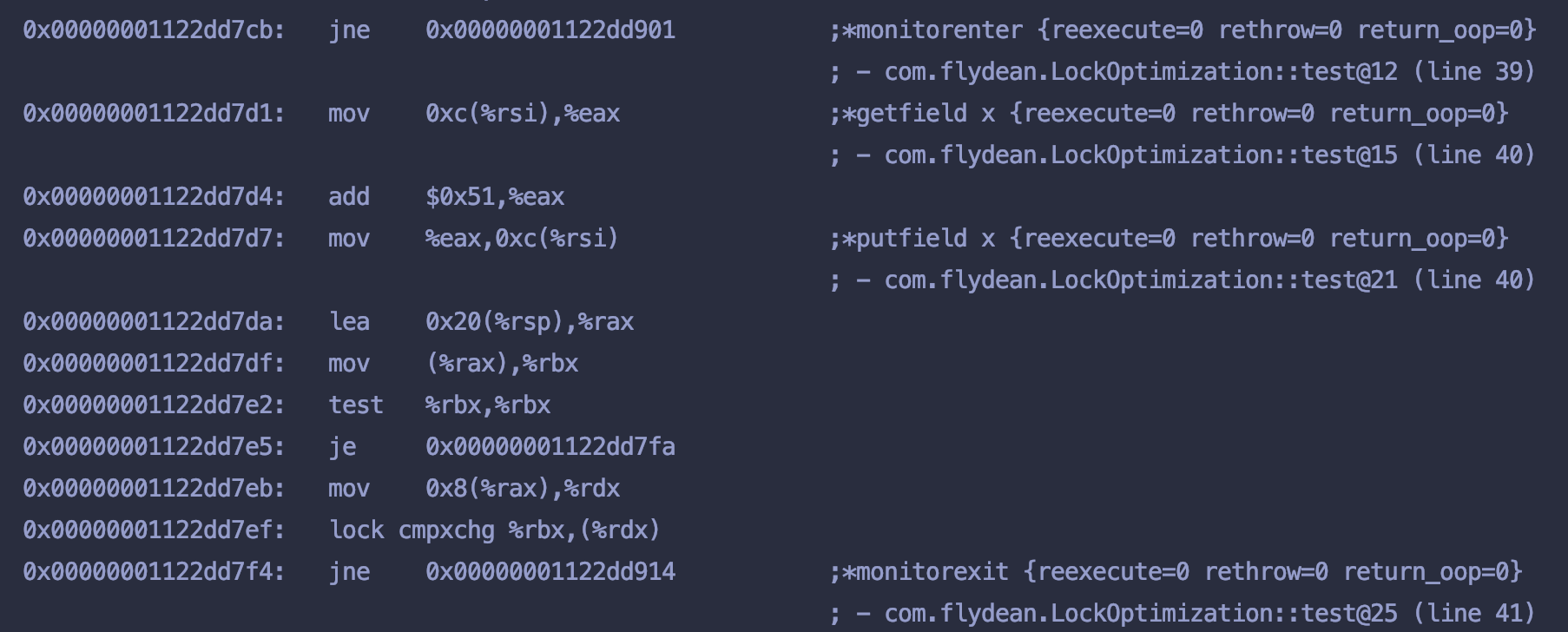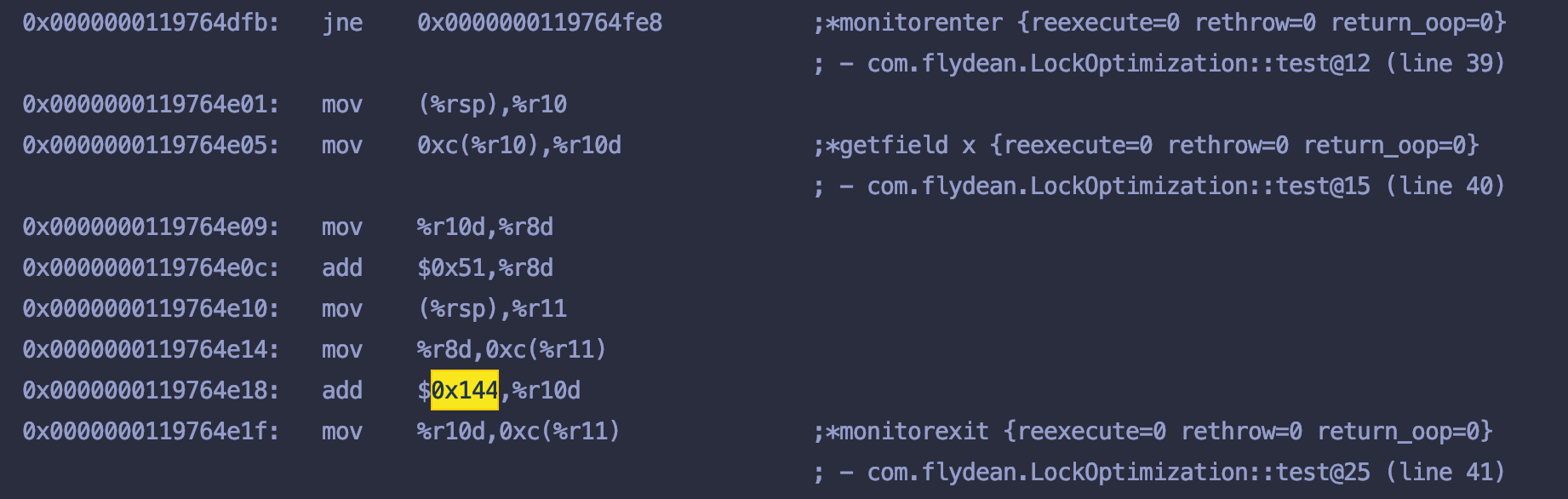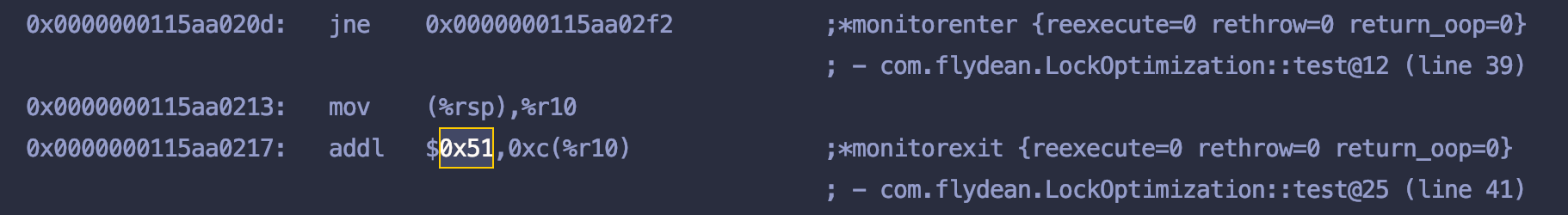# 循环展开和粗化锁

``````for (int i = 0; i < 1000; i++) {
x += 0x51;
}
``````

``````for (int i = 0; i < 250; i++) {
x += 0x144; //0x51 * 4
}
``````

``````for (int i = 0; i < 1000; i++) {
synchronized (this) {
x += 0x51;
}
}
``````

``````@Warmup(iterations = 10, time = 1, timeUnit = TimeUnit.SECONDS)
@Measurement(iterations = 5, time = 1, timeUnit = TimeUnit.SECONDS)
@Fork(value = 1,
jvmArgsPrepend = {
"-XX:-UseBiasedLocking",
"-XX:CompileCommand=print,com.flydean.LockOptimization::test"
}
)
@State(Scope.Benchmark)
@BenchmarkMode(Mode.AverageTime)
@OutputTimeUnit(TimeUnit.NANOSECONDS)
public class LockOptimization {

int x;
@Benchmark
@CompilerControl(CompilerControl.Mode.DONT_INLINE)
public void test() {
for (int i = 0; i < 1000; i++) {
synchronized (this) {
x += 0x51;
}
}
}

public static void main(String[] args) throws RunnerException {
Options opt = new OptionsBuilder()
.include(LockOptimization.class.getSimpleName())
.build();
new Runner(opt).run();
}
}
``````

# 分析Assembly日志``````Benchmark              Mode  Cnt     Score     Error  Units
LockOptimization.test  avgt    5  5601.819 ± 620.017  ns/op
``````

# 禁止Loop unrolling``````Benchmark              Mode  Cnt      Score      Error  Units
LockOptimization.test  avgt    5  20846.709 ± 3292.522  ns/op
``````